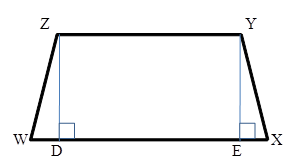Chapter 4.4, Problem 19E### Elementary Geometry for College St...

6th Edition
Daniel C. Alexander + 1 other
ISBN: 9781285195698

#### Solutions

Chapter
Section### Elementary Geometry for College St...

6th Edition
Daniel C. Alexander + 1 other
ISBN: 9781285195698
Textbook Problem
137 views

# In isosceles trapezoid WXYZ with bases Z Y ¯ and W X ¯ , ZY = 8, YX = 10 and WX = 20. Find height h (the length of Z D ¯ or Y E ¯ ).Exercises 19, 20

To determine

To Find:

The height of an isosceles trapezoid.

Explanation

Consider the following isosceles trapezoid.

Given: ZY = 8, YX = 10 and WX = 20.

In the ΔEXY, EX=WXZY2=2082=6.

According to Pythagoras theorem, (YX)2=

### Still sussing out bartleby?

Check out a sample textbook solution.

See a sample solution

#### The Solution to Your Study Problems

Bartleby provides explanations to thousands of textbook problems written by our experts, many with advanced degrees!

Get Started